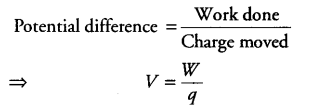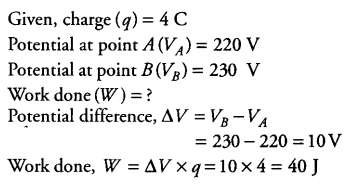# (i) State the relation between potential difference, work done and charge moved

(i) State the relation between potential difference, work done and charge moved.
(ii) Calculate the workdone in moving a charge of 4 C h m a point at 220 V to a point at 230 V.

The relation between potential difference, work done and charge moved is given by(ii)